# question from week 4 challenge(geometry) q's 17

• This is a question that involves counting triangles... and after reading through the explanation, here is what I understand: I know the meaning of y < x + x = 2x.

Here is what I don't understand: I am still confused about how can I know which section I don't need to count and which is the one that I need to count in.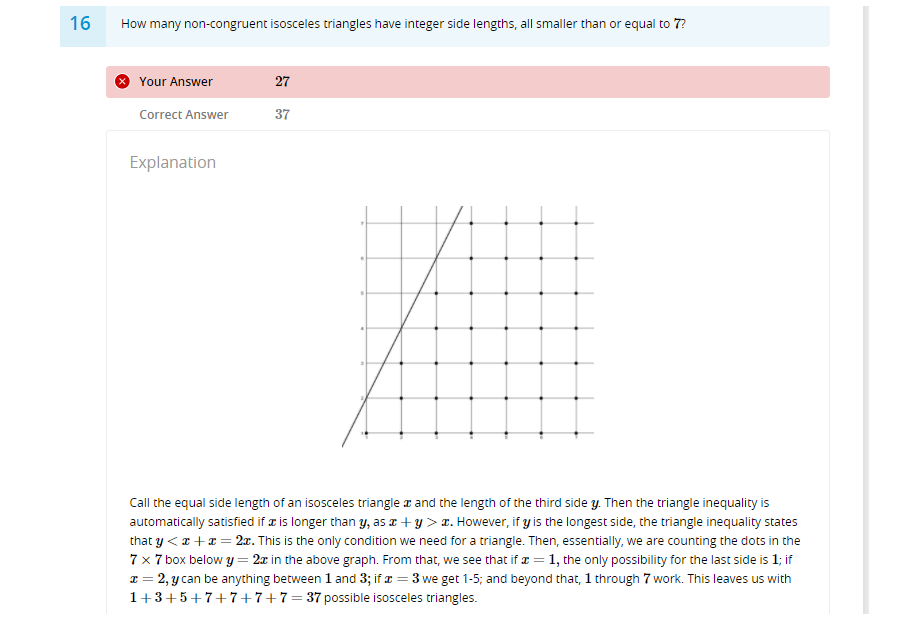• Hey @victorioussheep !

This is a really unique type of counting problem. The idea here is that we're gonna call the two lengths of the isoceles triangle $$x$$ and $$y$$. Since the triangle has side lengths less than or equal to 7, this means that $$x,y \leq 7$$. And, add that onto the triangle inequality, which says that $$y <2x$$ in this problem.

What we've done now is make three inequalities: $$x \leq 7, y \leq 7, y < 2x$$. So, if we graph them, that means that every point on the interior of the intersection of all three inequalities satisfies the conditions necessary in the problem!

Here's what each of the inequalities looks like individually: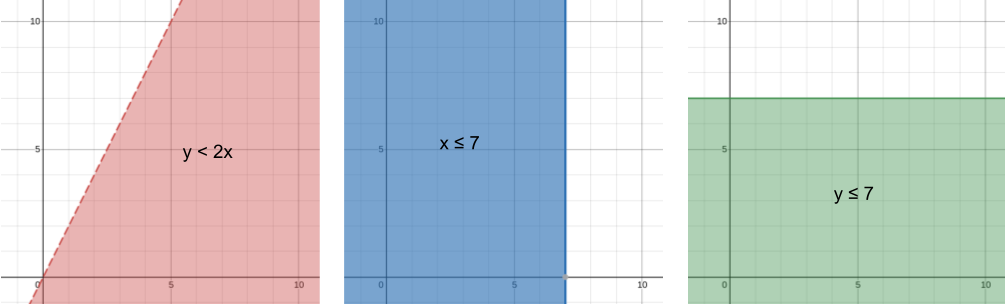For each of the graphs, if the region is shaded in, that means it satisfies that inequality. Dotted line means that it doesn't work, and a solid line means that it does work. If we wanted to interpret the graphs in terms of the problem, the red graph shows $$y<2x$$, which is all the points where side length $$y$$ is less than twice side length $$x$$. The blue graph shows all the points where side length $$x$$ is less than or equal to 7, and the green graph shows all the points where side length $$y$$ is less than or equal to 7.

So, since we want to find the triangles that satisfy ALL of the inequalities, let's graph all of them at once and see which points is shaded by all three colors.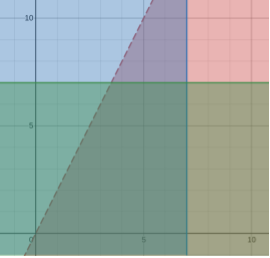The region where all three inequalities overlap is the part I shaded in white: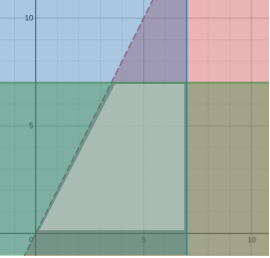I also forgot to mention that both $$x,y>0$$, but I think that's just a given. But do you see that all the points that fall within that trapezoidal region satisfy all three inequalities? That means we just have to count the points that fall in that region. Remember that we can't count the points on the dotted line, but we can count the ones on the solid lines (dotted line means $$<$$ or $$>$$, so it doesn't include that but solid lines, which are $$\leq$$ and $$\geq$$, do include the line)

So here are all the points I counted: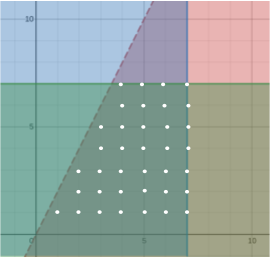which is $$\boxed{37}$$ in total.

I'm not sure how much you know already about graphing inequalities, so if this is confusing, let me know which parts you understood and which parts you want me to explain, and I can surely do that!

By the way, the graphing calculator I used is desmos.com. It's a great graphing calculator that's really useful in all types of math<img src="blob:chrome-untrusted://media-app/7912d715-7ff2-463a-8e27-b415d4e1f06a" alt="Untitled drawing (4).png"/>

• @quacker88
I can totally understand your method. It is actually easier for me to understand your strategy than the one that Professor Loh talks about, but that is because I prefer visual learning. However, there is a problem though - how can I tackle this question if I am in a competition? I probably don't have time to do draw it out.

• @victorioussheep Glad that you understood! Yeah I usually prefer visual representations too.

Very good point that you bring up: it's usually not very convenient to just pull out a graphing calculator. In that case, I think I would just list out all the possible cases without thinking about variables. Since the sides are at most 7, it's relatively simple casework.

If two congruent (same) sides have length 1, then the last side must be length 1.

If the congruent sides have length 2, then the last side must be 1, 2, or 3. Doing this all the way up to 7, we have

sides 1, 1: last side can only 1
sides 2, 2: last side can only be 1, 2, 3
sides 3, 3: last side can only be 1, 2, 3, 4, 5
sides 4, 4: last side can only be 1, 2, 3, 4, 5, 6, 7
sides 5, 5: last side can only be 1, 2, 3, 4, 5, 6, 7 (remember, sides can only be at most length 7!)
sides 6, 6: last side can only be 1, 2, 3, 4, 5, 6, 7
sides 7, 7: last side can only be 1, 2, 3, 4, 5, 6, 7

Adding them up gives you $$1+3+5+7+7+7+7=37$$.
Honestly, this is probably the fastest and easiest way to do it, and it works for larger numbers because you can see that there's a pattern pretty quickly. Notice how if there was no limit on the length of the third side, it would have kept going to the next odd number (what I mean here is that, normally a triangle with sides 5, 5 could have the last length being anywhere from 1 to 9. sides 6, 6 would have 1 to 11. It keeps going up to the next odd number).

So, I think the takeaway for these types of problems is usually to try to do some sort of casework, it usually won't fail you. And, try to find a pattern on top of that so it's easier to add up at the endGraphing is just another way to do the same problem. Although it's probably slower to graph for this specific scenario, there are plenty of questions where graphing is very useful, so it's a good idea to learn how to use it!

• @quacker88
ok, thanks! now I think I am able to complete this problem without do all these graphing. I figure that when a question asks you to do some counting, there is a 99% that there will be somewhat a pattern in between them...

• @victorioussheep Yeah exactly! Always keep your eyes peeled for patterns when doing counting problems